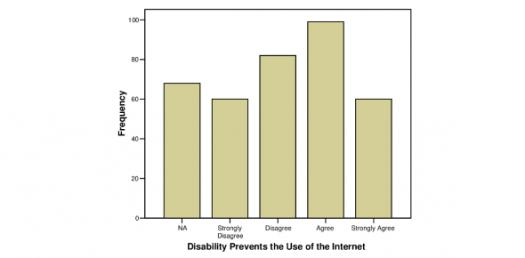Table And Bar Graph Test! Trivia Questions Quiz

12 Questions | Total Attempts: 1493Settings.

• 1.
Study the following table and answer the questions. Number of Candidates Appeared and Qualified in a Competitive Examination from the Different States Over the Years. -  The total number of candidates qualified from all the states together in 1997 is approximately what percentage of the total number of candidates qualified from all the states together in 1998?
• A.

72%

• B.

77%

• C.

80%

• D.

83%

• 2.
In which of the given years the number of candidates appeared from State P has maximum percentage of qualified candidates?
• A.

1997

• B.

1998

• C.

1999

• D.

2001

• 3.
What is the average candidates who appeared from State Q during the given years?
• A.

8700

• B.

8760

• C.

8990

• D.

8920

• 4.
What is the percentage of candidates qualified from State N for all the years together, over the candidates appeared from State N during all the years together?
• A.

12.36%

• B.

12.16%

• C.

11.47%

• D.

11.15%

• 5.
The percentage of total number of qualified candidates to the total number of appeared candidates among all the five states in 1999 is?
• A.

11.49%

• B.

11.84%

• C.

12.21%

• D.

12.57%

• 6.
Combining the states P and Q together in 1998, what is the percentage of the candidates qualified to that of the candidate appeared?
• A.

10.87%

• B.

11.49%

• C.

12.35%

• D.

12.54%

• 7.
The bar graph given below shows the data of the production of paper (in lakh tonnes = One Lakh is equal to One Hundred Thousand (100,000)) by three different companies X, Y and Z over the years.   For which of the following years, the percentage rise/fall in production from the previous year is the maximum for Company Y?
• A.

1997

• B.

1998

• C.

1999

• D.

2000

• 8.
What is the ratio of the average production of Company X in the period 1998-2000 to the average production of Company Y in the same period?
• A.

1:1

• B.

15:17

• C.

23:25

• D.

27:29

• 9.
The average production for five years was maximum for which company?
• A.

X

• B.

Y

• C.

Z

• D.

X and Z both

• 10.
In which year was the percentage of production of Company Z to the production of Company Y the maximum?
• A.

1996

• B.

97

• C.

98

• D.

99

• 11.
What is the percentage increase in the production of Company Y from 1996 to 1999?
• A.

30%

• B.

45%

• C.

50%

• D.

60%

• 12.
What is the difference between the production of Company Z in 1998 and Company Y in 1996?
• A.

2,00,000 tons

• B.

20,00,000 tons

• C.

20,000 tons

• D.

2,00,00,000 tons

Related TopicsBack to top